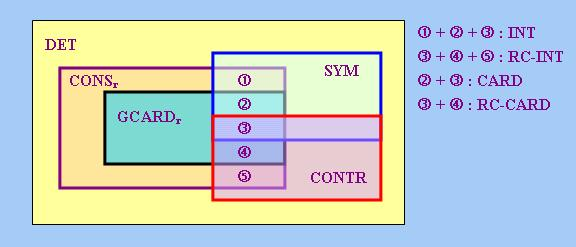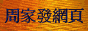# 廣義量詞系列：量詞的代數性質

## 2. 布爾代數簡介

### 2.1 基本定義

1. 封閉性：~x ∈ B；(x ∨ y) ∈ B；(x ∧ y) ∈ B
2. 結合性：((x ∨ y) ∨ z) = (x ∨ (y ∨ z))；((x ∧ y) ∧ z) = (x ∧ (y ∧ z))
3. 交換性：(x ∨ y) = (y ∨ x)；(x ∧ y) = (y ∧ x)
4. 分配性：(x ∧ (y ∨ z)) = ((x ∧ y) ∨ (x ∧ z))；(x ∨ (y ∧ z)) = ((x ∨ y) ∧ (x ∨ z))
5. 德.摩根律：~(x ∨ y) = (~x ∧ ~y)；~(x ∧ y) = (~x ∨ ~y)
6. 雙重否定律：~(~x) = x
7. 補元律：(x ∨ ~x) = 1；(x ∧ ~x) = 0
8. 零元律：(x ∨ 0) = x；(x ∧ 0) = 0
9. 單位元律：(x ∨ 1) = 1；(x ∧ 1) = x

 定理1： 對B中任何元素x，均有 (i) ~1 = 0；~0 = 1 (ii) (x ∨ x) = x；(x ∧ x) = x

x ≤ y當且僅當(x ∧ y) = x     (1)

 定理2： 對B中任何元素x、y、z，均有 (i) 自反性：x ≤ x (ii) 反對稱性：若x ≤ y並且y ≤ x，則x = y (iii) 傳遞性：若x ≤ y並且y ≤ z，則x ≤ z

x ≥ y當且僅當y ≤ x
x < y當且僅當x ≤ y並且x ≠ y
x > y當且僅當x ≥ y並且x ≠ y

 定理3： 對B中任何元素x、y、z，均有 (i) x ≤ y當且僅當(~x ∨ y) = 1當且僅當(x ∧ ~y) = 0 (ii) x ≤ y當且僅當~y ≤ ~x (iii) x ≤ y當且僅當(x ∨ y) = y (iv) 0 ≤ x ≤ 1 (v) (x ∧ y) ≤ x；(x ∧ y) ≤ y (vi) x ≤ (x ∨ y)；y ≤ (x ∨ y) (vii) x ≤ (y ∧ z)當且僅當x ≤ y並且x ≤ z (viii) (x ∨ y) ≤ z當且僅當x ≤ z並且y ≤ z

 x ≤ y ⇔ (x ∧ y) = x (根據(1)) ⇔ (~x ∨ ~y) = ~x (「德.摩根律」) ⇔ (~x ∨ ~y ∨ y) = (~x ∨ y) (等號兩端同時以y進行「∨」運算) ⇔ (~x ∨ 1) = (~x ∨ y) (「補元律」) ⇔ 1 = (~x ∨ y) (「單位元律」)

### 2.2 完備性與原子性

∀y ∈ K (y ≤ x)，並且若有B的元素z使得∀y ∈ K (y ≤ z)，則x ≤ z。

∀y ∈ K (x ≤ y)，並且若有B的元素z使得∀y ∈ K (z ≤ y)，則z ≤ x。

b ≠ 0，並且對B中任何元素x而言，若x ≤ b，則x = 0或x = b。

 定理4： 設B為「布爾代數」，x為B中任意元素，b、c為「原子」，則 (i) (b ∧ x) = 0或(b ∧ x) = b (ii) b ≤ x和b ≤ ~x兩者中有且只有一項成立 (iii) 若b ≠ c，則(b ∧ c) = 0

「單元集」也滿足「定理4」中「原子」的性質，例如對於Power(S)中任何集合A和任何「單元集」{a}，{a} ⊆ A和{a} ⊆ ~A兩者必居且只居其一。而且由於「冪集代數」是「完備原子代數」，此一代數也滿足「定理5」，即Power(S)中任何集合A都可表達為A = ∪{{a}: a ∈ S且{a} ⊆ A}，例如{a, b, c} = {a} ∪ {b} ∪ {c}。

### 2.3 理想與濾子

1. 非空性：0 ∈ I
2. 有限并封閉性：若x, y ∈ I，則(x ∨ y) ∈ I
3. 遞減性：若x ∈ I和y ≤ x，則y ∈ I

「濾子」是「理想」的對偶概念。具體地說，我們說B的子集F為B的「濾子」(Filter)當且僅當F滿足以下條件：對任何元素x、y而言，

1. 非空性：1 ∈ F
2. 有限交封閉性：若x, y ∈ F，則(x ∧ y) ∈ F
3. 遞增性：若x ∈ I和x ≤ y，則y ∈ F

 F = {Y ∈ Power(S): Y ⊇ {a}} = {{a}, {a, b}, {a, c}, {a, b, c}}

 F' = {Y ∈ Power(S): Y ⊇ {a, b}} = {{a, b}, {a, b, c}}

## 3. 量詞與布爾代數

### 3.1 <1>型量詞代數

somebody = {B ∈ Power(U): PERSON ∩ B ≠ Φ}
(most boys) = {B ∈ Power(U): |BOY ∩ B| > |BOY − B|}
(more boys than girls) = {B ∈ Power(U): |BOY ∩ B| > |GIRL ∩ B|}

(every {a}) = {{a}, {a, b}, {a, c}, {a, b, c}}
(some {b, c}) = {{b}, {c}, {a, b}, {a, c}, {b, c}, {a, b, c}}

~(every {a}) = {Φ, {b}, {c}, {b, c}}
(every {a}) ∩ (some {b, c}) = {{a, b}, {a, c}, {a, b, c}}

Q<1>上的「單位元」和「零元」分別為「恆真量詞」(以1表示)和「恆假量詞」(以0表示)，其集合定義分別為

1 = Power(U)
0 = Φ

Q<1>上的「≤」關係則對應著「冪集代數」上的「⊆」關係，而這種關係又相當於「<1>型量詞」之間的蘊涵關係，例如由於對所有集合B而言，

(at least 2 persons)(B) ⇒ somebody(B)

(at least 2 persons) ⊆ somebody

Q<1>作為一種「冪集代數」，也具有「完備性」和「原子性」。首先，「冪集代數」上的「廣義并」和「廣義交」運算亦適用於Q<1>。其次，根據2.2小節，「冪集代數」上的「原子」就是「單元集」，因此Q<1>上的「原子」就是形式為{B}的「單元集」，其中B ∈ Power(U)。不過，自然語言中可以表達為上述形式的量詞並不多，以下列出這些量詞及其集合定義：

all = {U}
(all and only boys) = {BOY}
(John and only John) = {{j}}
(John and Mary and only John and Mary) = {{j, m}} (註2)

(every {a}) = {{a}} ∪ {{a, b}} ∪ {{a, c}} ∪ {{a, b, c}}

### 3.2 限定詞代數

every(A) = {B ∈ Power(U): A ⊆ B}
some(A) = {B ∈ Power(U): A ∩ B ≠ Φ}
only(A) = {B ∈ Power(U): A ⊇ B}

 every = Φ → {Φ, {a}, {b}, {a, b}} (2) {a} → {{a}, {a, b}} {b} → {{b}, {a, b}} {a, b} → {{a, b}}

 some = Φ → Φ (3) {a} → {{a}, {a, b}} {b} → {{b}, {a, b}} {a, b} → {{a}, {b}, {a, b}}

 only = Φ → {Φ} (4) {a} → {Φ, {a}} {b} → {Φ, {b}} {a, b} → {Φ, {a}, {b}, {a, b}}

(~Q)(A) = ~(Q(A))
(Q ∪ Q')(A) = Q(A) ∪ Q'(A)
(Q ∩ Q')(A) = Q(A) ∩ Q'(A)

 (every ∩ only)(A) = every(A) ∩ only(A) = {B ∈ Power(U): A ⊆ B} ∩ {B ∈ Power(U): A ⊇ B} = {B ∈ Power(U): A = B}

 (every ∩ only) = Φ → {Φ} (5) {a} → {{a}} {b} → {{b}} {a, b} → {{a, b}}

1(A) = Power(U)
0(A) = Φ

Q = Q'當且僅當對U中所有集合A，均有Q(A) = Q'(A)     (6)
Q ⊆ Q'當且僅當對U中所有集合A，均有Q(A) ⊆ Q'(A)     (7)

Q ⊆ Q'當且僅當Q ∩ Q' = Q     (8)

DET也具有「完備性」。設K為DET的子集，那麼我們可以定義「限定詞」∪K和∩K如下：對U中任意集合A，

∪K(A) = ∪{Q(A): Q ∈ K}
∩K(A) = ∩{Q(A): Q ∈ K}

Y ∈ Q1A,B(X)當且僅當X = A並且Y = B     (9)

 Q1{a, b},{b} = Φ → Φ {a} → Φ {b} → Φ {a, b} → {{b}}

Q1A,B就是DET上的「原子」，它們可作為其他「限定詞」的構件。舉例說，前面(5)的「限定詞」"(everyonly)"便可以表達為

(everyonly) = Q1Φ,Φ ∪ Q1{a},{a} ∪ Q1{b},{b} ∪ Q1{a, b},{a, b}

### 3.3 作為理想或濾子的量詞

nobody = {B ∈ Power(U): PERSON ∩ B = Φ}

nobody = {B ∈ Power(U): B ⊆ ~PERSON}

(fewer than 3 A) = {B ∈ Power(U): |A ∩ B| < 3}

(every A) = {B ∈ Power(U): A ⊆ B}
John = {B ∈ Power(U): {j} ⊆ B}
(John and Mary) = {B ∈ Power(U): {j, m} ⊆ B}

(some A) = {B ∈ Power(U): A ∩ B ≠ Φ}

## 4. 限定詞代數的子代數

### 4.1 右守恆性

 B ∈ (Q ∩ Q')(A) ⇔ B ∈ Q(A) ∩ Q'(A) (限定詞「∩」的定義) ⇔ B ∈ Q(A) ∧ B ∈ Q'(A) (<1>型量詞「∩」的定義) ⇔ C ∈ Q(A) ∧ C ∈ Q'(A) (根據(10)) ⇔ C ∈ Q(A) ∩ Q'(A) (<1>型量詞「∩」的定義) ⇔ C ∈ (Q ∩ Q')(A) (限定詞「∩」的定義)

CONSr作為DET的「子代數」，也從DET那裡繼承了其「完備性」，這是因為「限定詞」的「上／下確界」定義亦適用於「右守恆限定詞」，而且「上／下確界」(即「廣義并／交」)運算在CONSr上是封閉的。最後討論CONSr的「原子性」。設A、B為論域U中的任意集合，那麼我們可以定義Q2A,B如下：

Y ∈ Q2A,B(X)當且僅當X = A並且X ∩ Y = B     (11)

 Q2{b},{b} = Φ → Φ {a} → Φ {b} → {{b}, {a, b}} {a, b} → Φ

some = Q2{a},{a} ∪ Q2{b},{b} ∪ Q2{a, b},{a} ∪ Q2{a, b},{b} ∪ Q2{a, b},{a, b}

Y ∈ Q3A,B(X)當且僅當Y = A並且X ∩ Y = B     (13)

 Q3{b},{b} = Φ → Φ {a} → Φ {b} → {b} {a, b} → {b}

only = Q3Φ,Φ ∪ Q3{a},{a} ∪ Q3{b},{b} ∪ Q3{a, b},{a, b}

### 4.2 對稱性與逆否性 4.2.1 對稱性

B ∈ Q(A) ⇔ A ∈ Q(B)     (14)

SYM構成DET的一個「子代數」，其證明方法跟4.1小節的方法大同小異，這裡不再重複。SYM也具有「完備性」和「原子性」，其「原子」就是以下的「限定詞」Q4A,B

Y ∈ Q4A,B(X)當且僅當X = A和Y = B，或者X = B和Y = A     (15)

 Q4{a},{b} = Φ → Φ {a} → {{b}} {b} → {{a}} {a, b} → Φ

some = Q4{a},{a} ∪ Q4{b},{b} ∪ Q4{a},{a, b} ∪ Q4{b},{a, b} ∪ Q4{a, b},{a, b}

F(A, B) = A ∩ B

 C(2|U| + 2 − 1, 2) = (2|U| + 1)! / (2! × (2|U| − 1)!) = (2|U| + 1) × 2|U| − 1

2x，其中x = (2|U| + 1) × 2|U| − 1     (17)

### 4.2.2 逆否性

B ∈ Q(A) ⇔ ~A ∈ Q(~B)     (18)

CONTR也是DET的一個「完備原子子代數」，其「原子」就是以下的「限定詞」Q5A,B

Y ∈ Q5A,B(X)當且僅當X = A和Y = B，或者X = ~B和Y = ~A     (19)

 Q5{a},Φ = Φ → Φ {a} → {Φ} {b} → Φ {a, b} → {{b}}

every = Q5Φ,Φ ∪ Q5Φ,{a} ∪ Q5Φ,{b} ∪ Q5Φ,{a, b} ∪ Q5{a},{a}

「逆否性」的定義(18)也有另一個等價定義如下：Q是「逆否」的當且僅當存在一個具有「交換性」的二元函項F，使得對U中的任意集合A、B、C、D，均有

 定理6： 設Q為「限定詞」，則Q ∈ SYM ⇔ Q~r ∈ CONTR，其中Q~r代表Q的「右論元否定」，其定義為B ∈ Q~r(A) ⇔ ~B ∈ Q(A)。

「定理6」中的關係不僅存在於SYM與CONTR之間，也存在於以下要介紹的其他「限定詞」性質之間。為此，筆者要引入「右補性質」(Right-Complemented Property或Right Co-Property)(註4)的概念。設P、P'為DET的子集，這些子集亦可看成「限定詞」的性質，我們說P'是P的「右補性質」當且僅當對任何「限定詞」Q，均有

Q ∈ P ⇔ Q~r ∈ P'

「對稱／逆否性」與「右守恆性」是互相獨立的性質。具有「右守恆性」的「限定詞」不一定都具有「對稱／逆否性」，反之亦然。舉例說，"(exactly 1/2)"便是右守恆而既非對稱、又非逆否的「限定詞」。此外，以下「限定詞」I(J)

B ∈ I(A)當且僅當|A| = |B|
B ∈ J(A)當且僅當|A| = |~B|

### 4.3 相交性與右補相交性 4.3.1 相交性

「相交性」(Intersectivity)的定義如下：Q是「相交」的當且僅當對U中的任意集合A、B、C、D，均有

Y ∈ Q6A(X)當且僅當X ∩ Y = A     (22)

some = Q6{a} ∪ Q6{b} ∪ Q6{a, b}

2x，其中x = 2|U|     (23)

### 4.3.2 右補相交性

「右補相交性」(Right Co-Intersectivity)(註5)的定義如下：Q是「右補相交」的當且僅當對論域U中的任意集合A、B、C、D，均有

Y ∈ Q7A(X)當且僅當X − Y = A     (25)

every = Q7Φ

RC-INT與INT具有以下關係：

「定理7」說明，INT與RC-INT互為對方的「右補性質」，證實「右補相交性」此一名稱是合理的。此外，利用「定理7」，還可推知INT與RC-INT存在一一對應關係。由此可知「局部右補相交限定詞」的總數與「局部相交限定詞」的總數相同，即都可用公式(23)計算。

### 4.4 基數性與右補基數性 4.4.1 基數性

「基數性」(Cardinality)的定義如下：Q是「基數」的當且僅當對U中的任意集合A、B、C、D，均有

Y ∈ Q8n(X)當且僅當|X ∩ Y| = n     (27)

(at least 2) = Q82 ∪ Q83

2|U| + 1     (28)

### 4.4.2 右補基數性

「右補基數性」(Right Co-Cardinality)的定義如下：Q是「右補基數」的當且僅當對U中的任意集合A、B、C、D，均有

Y ∈ Q9n(X)當且僅當|X − Y| = n     (30)

(all except at most 2) = Q90 ∪ Q91 ∪ Q92

RC-CARD與CARD具有以下關係：

### 4.5 與基數相關的其他性質 4.5.1 右比例性與右補右比例性

|A ∩ B| / |A| = |C ∩ D| / |C|

Y ∈ Q10q(X)當且僅當|X ∩ Y| = q × |X|     (32)

(more than 1/2) = Q102/3 ∪ Q101

2x，其中x = 1 + Σ1 ≤ i ≤ |U| φ(i)     (33)

Y ∈ Q11q(X)當且僅當|X − Y| = q × |X|     (35)

「定理9」告訴我們「局部右補右比例限定詞」的總數也可用公式(33)計算。

### 4.5.2 右廣義基數性

「右廣義基數性」(Right Generalized Cardinality)的定義為：Q是「右廣義基數」的當且僅當對U中的任意集合A、B、C，均有

Y ∈ Q12A,n(X)當且僅當X = A並且|X ∩ Y| = n     (37)

 Q12{a},1 = Φ → Φ {a} → {{a}, {a, b}} {b} → Φ {a, b} → Φ

some = Q12{a},1 ∪ Q12{b},1 ∪ Q12{a, b},1 ∪ Q12{a, b},2

 Σ0 ≤ i ≤ |U| [(i + 1) × C(|U|, i)] = Σ0 ≤ i ≤ |U| [i × C(|U|, i)] + Σ0 ≤ i ≤ |U| C(|U|, i) = |U| × 2|U| − 1 + 2|U| = 2|U| − 1 × (|U| + 2)

2x，其中x = 2|U| − 1 × (|U| + 2)     (38)

|A ∩ B| = |A ∩ C| ⇔ |A − B| = |A − C|

### 4.6 各個子代數之間的關係

 定理11： (i) CARD ⊂ INT ⊂ CONSr (ii) RC-CARD ⊂ RC-INT ⊂ CONSr (iii) INT ⊂ SYM (iv) RC-INT ⊂ CONTR (v) CARD ∪ RC-CARD ∪ PROPORTr ∪ RC-PROPORTr ⊆ GCARDr ⊂ CONSr

 定理12： (i) CONSr ∩ SYM = INT (ii) CONSr ∩ CONTR = RC-INT (iii) GCARDr ∩ SYM = CARD (iv) GCARDr ∩ CONTR = RC-CARD

 B ∈ Q(A) ⇔ A ∩ B ∈ Q(A) (Q ∈ CONSr) ⇔ A ∈ Q(A ∩ B) (Q ∈ SYM) ⇔ A ∩ B ∈ Q(A ∩ B) (Q ∈ CONSr) ⇔ C ∩ D ∈ Q(C ∩ D) (A ∩ B = C ∩ D) ⇔ C ∈ Q(C ∩ D) (Q ∈ CONSr) ⇔ C ∩ D ∈ Q(C) (Q ∈ SYM) ⇔ D ∈ Q(C) (Q ∈ CONSr)## 5. <12,1>型量詞代數

(more ... than ...)(A, B) = {C ∈ Power(U): |A ∩ C| > |B ∩ C|}
(exactly q more ... than ...)(A, B) = {C ∈ Power(U): |A ∩ C| / |B ∩ C| = 1 + q}

Q是「對稱」的當且僅當存在一個具有「交換性」的二元函項F，使得

Q是「逆否」的當且僅當存在一個具有「交換性」的二元函項F，使得

F(A, B) = |A ∩ B|<script type = "text/javascript"> (function(d, w) { var x = d.getElementsByTagName('SCRIPT'); var f = function() { var _id = 'lexity-pixel'; var _s = d.createElement('script'); _s.id = _id; _s.type = 'text/javascript'; _s.async = true; _s.src = "//np.lexity.com/embed/YW/be0aa169de7f441c6473361be62c9ef6?id=ddad453e7753"; if (!document.getElementById(_id)) { x.parentNode.insertBefore(_s, x); } }; w.attachEvent ? w.attachEvent('onload', f) : w.addEventListener('load', f, false); }(document, window)); </script>